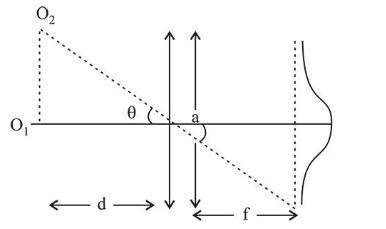# The aperture diameter of aelescope is 5 mQuestion:

The aperture diameter of aelescope is $5 \mathrm{~m}$. The separation between the moon and the earth is $4 \times 10^{5} \mathrm{~km}$. With light of wavelength of $5500 A$, the minimum separation between objects on the surface of moon, so that they are just resolved, is close to:

1. (1) $60 \mathrm{~m}$

2. (2) $20 \mathrm{~m}$

3. (3) $200 \mathrm{~m}$

4. (4) $600 \mathrm{~m}$

Correct Option: 1

Solution:

(1)Smallest angular separation between two distant objects here moon and earth,

$\theta=1.22 \frac{\lambda}{a}$

$\mathrm{a}=$ aperture diameter of telescope

Distance $\mathrm{O}_{1} \mathrm{O}_{2}=(\theta) d$

Minimum separation between objects on the surface of moon,

$=\left(1.22 \frac{\lambda}{a}\right) d$

$=\frac{(1.22)\left(5500 \times 10^{-10}\right) \times 4 \times 10^{5} \times 10^{3}}{5}$

$=5368 \times 10^{-2} \mathrm{~m}=53.68 \mathrm{~m} \approx 60 \mathrm{~m}$